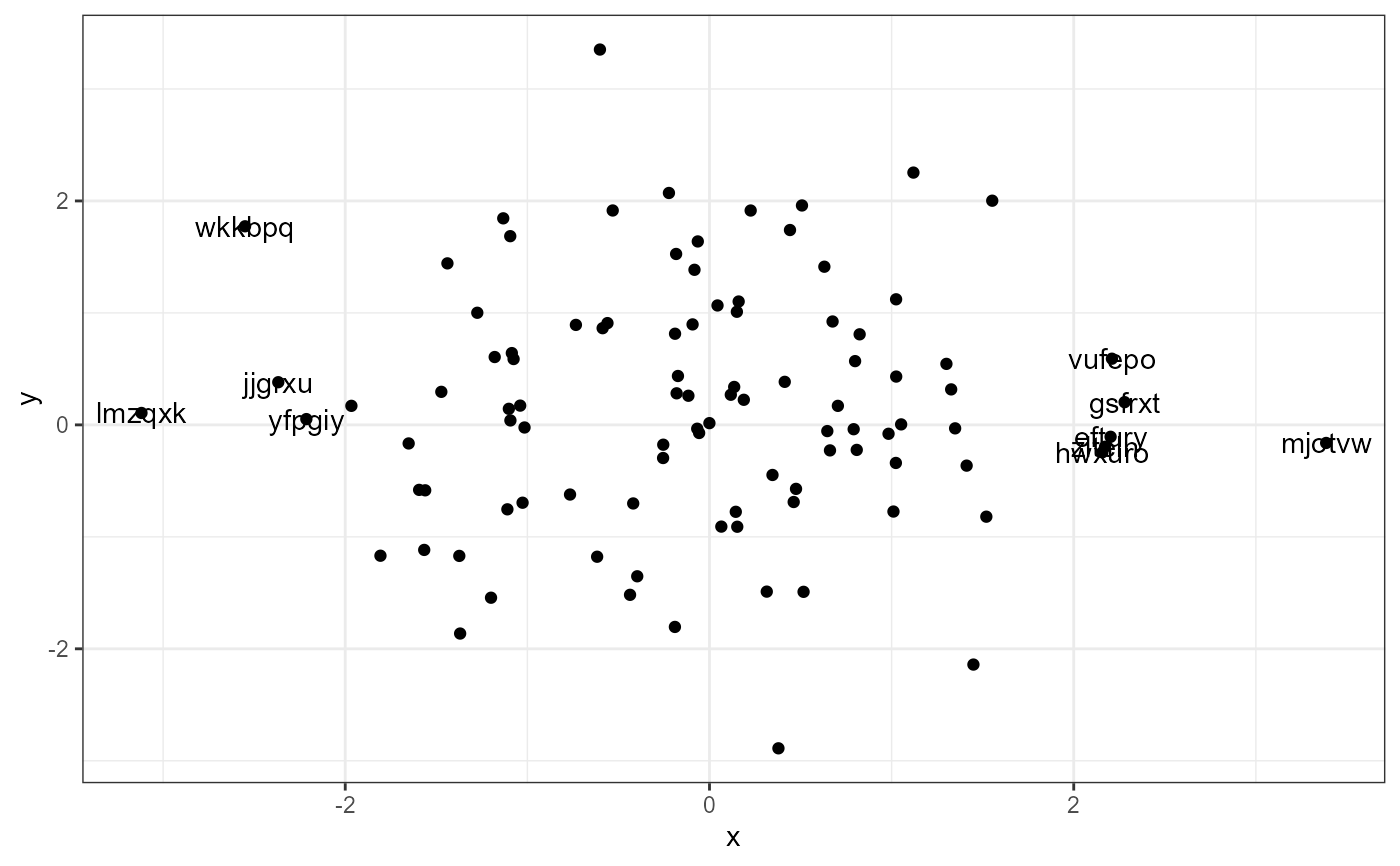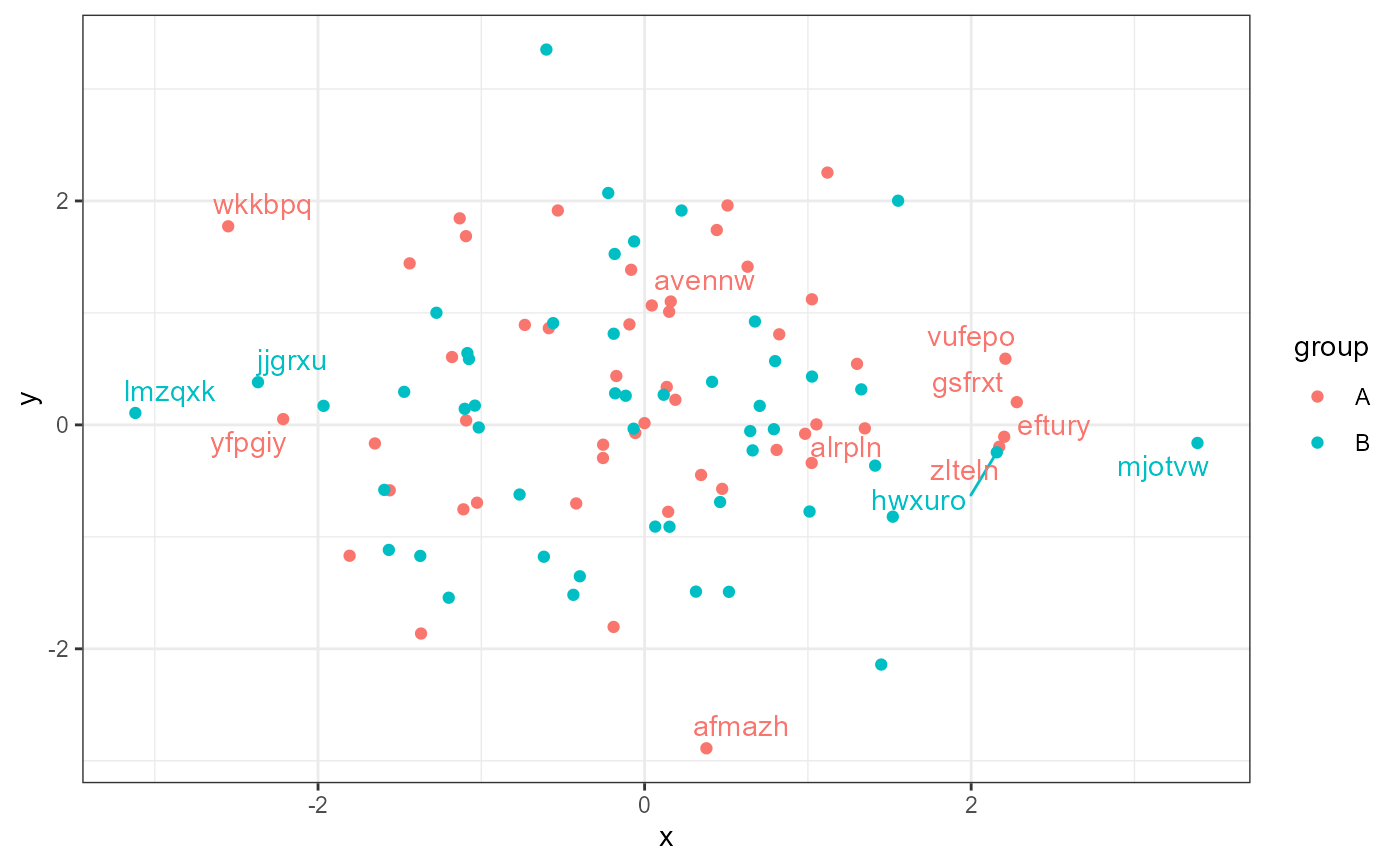stat_dens1d_labels() Sets values mapped to the label aesthetic to "" or a user provided character string based on the local density in regions of a plot panel. Its main use is together with repulsive geoms from package ggrepel to restrict labeling to the low density tails of a distribution. By default the data are handled all together, but it is also possible to control labeling separately in each tail.

If there is no mapping to label in data, the mapping is set to rownames(data), with a message.

## Usage

stat_dens1d_labels(
mapping = NULL,
data = NULL,
geom = "text",
position = "identity",
...,
keep.fraction = 0.1,
keep.number = Inf,
keep.sparse = TRUE,
keep.these = FALSE,
exclude.these = FALSE,
these.target = "label",
pool.along = c("x", "none"),
xintercept = 0,
invert.selection = FALSE,
bw = "SJ",
kernel = "gaussian",
n = 512,
orientation = c("x", "y"),
label.fill = "",
return.density = FALSE,
na.rm = TRUE,
show.legend = FALSE,
inherit.aes = TRUE
)

## Arguments

mapping

The aesthetic mapping, usually constructed with aes or aes_. Only needs to be set at the layer level if you are overriding the plot defaults.

data

A layer specific dataset - only needed if you want to override the plot defaults.

geom

The geometric object to use display the data.

position

The position adjustment to use for overlapping points on this layer

...

other arguments passed on to layer. This can include aesthetics whose values you want to set, not map. See layer for more details.

keep.fraction

numeric vector of length 1 or 2 [0..1]. The fraction of the observations (or rows) in data to be retained.

keep.number

integer vector of length 1 or 2. Set the maximum number of observations to retain, effective only if obeying keep.fraction would result in a larger number.

keep.sparse

logical If TRUE, the default, observations from the more sparse regions are retained, if FALSE those from the densest regions.

keep.these, exclude.these

character vector, integer vector, logical vector or function that takes one or more variables in data selected by these.target. Negative integers behave as in R's extraction methods. The rows from data indicated by keep.these and exclude.these are kept or excluded irrespective of the local density.

these.target

character, numeric or logical selecting one or more column(s) of data. If TRUE the whole data object is passed.

pool.along

character, one of "none" or "x", indicating if selection should be done pooling the observations along the x aesthetic, or separately on either side of xintercept.

xintercept

numeric The split point for the data filtering.

invert.selection

logical If TRUE, the complement of the selected rows are returned.

bw

numeric or character The smoothing bandwidth to be used. If numeric, the standard deviation of the smoothing kernel. If character, a rule to choose the bandwidth, as listed in bw.nrd.

kernel

character See density for details.

numeric A multiplicative bandwidth adjustment. This makes it possible to adjust the bandwidth while still using the a bandwidth estimator through an argument passed to bw. The larger the value passed to adjust the stronger the smoothing, hence decreasing sensitivity to local changes in density.

n

numeric Number of equally spaced points at which the density is to be estimated for applying the cut point. See density for details.

orientation

character The aesthetic along which density is computed. Given explicitly by setting orientation to either "x" or "y".

label.fill

character vector of length 1 or a function.

return.density

logical vector of lenght 1. If TRUE add columns "density" and "keep.obs" to the returned data frame.

na.rm

a logical value indicating whether NA values should be stripped before the computation proceeds.

show.legend

logical. Should this layer be included in the legends? NA, the default, includes if any aesthetics are mapped. FALSE never includes, and TRUE always includes.

inherit.aes

If FALSE, overrides the default aesthetics, rather than combining with them. This is most useful for helper functions that define both data and aesthetics and shouldn't inherit behaviour from the default plot specification, e.g. borders.

## Value

A plot layer instance. Using as output data the input

data after value substitution based on a 1D the filtering criterion.

## Details

stat_dens1d_labels() is designed to work together with geometries from package 'ggrepel'. To avoid text labels being plotted over unlabelled points the corresponding rows in data need to be retained but labels replaced with the empty character string, "". Function stat_dens1d_filter cannot be used with the repulsive geoms from 'ggrepel' because it drops the observations.

stat_dens1d_labels() can be useful also in other situations, as the substitution character string can be set by the user by passing an argument to label.fill. If this argument is NULL the unselected rows are filtered out.

The local density of observations along x or y is computed with function density and used to select observations, passing to the geom all the rows in its data input but with with the text of labels replaced in those "not kept". The default is to select observations in sparse regions of the plot, but the selection can be inverted so that only observations in the densest regions are returned. Specific observations can be protected from having the label replaced by passing a suitable argument to keep.these. Logical and integer vectors function as indexes to rows in data, while a character vector is compared to values in the variable mapped to the label aesthetic. A function passed as argument to keep.these will receive as argument the values in the variable mapped to label and should return a character, logical or numeric vector as described above.

How many labels are retained intact in addition to those in keep.these is controlled with arguments passed to keep.number and keep.fraction. keep.number sets the maximum number of observations selected, whenever keep.fraction results in fewer observations selected, it is obeyed. If xintercept is a finite value within the x range of the data and pool.along is passed "none" the data are split into two groups and keep.number and keep.fraction are applied separately to each tail with density still computed jointly from all observations. If the length of keep.number and keep.fraction is one, half this value is used each tail, if their length is two, the first value is use for the left tail and the second value for the right tail (or if using orientation = "y" the lower and upper tails, respectively).

Computation of density and of the default bandwidth require at least two observations with different values. If data do not fulfill this condition, they are kept only if keep.fraction = 1. This is correct behavior for a single observation, but can be surprising in the case of multiple observations.

Parameters keep.these and exclude.these make it possible to force inclusion or exclusion of labels after the density is computed. In case of conflict, exclude.these overrides keep.these.

## Note

Which points are kept and which not depends on how dense and flexible is the density curve estimate. This depends on the values passed as arguments to parameters n, bw and kernel. It is also important to be aware that both geom_text() and geom_text_repel() can avoid overplotting by discarding labels at the plot rendering stage, i.e., what is plotted may differ from what is returned by this statistic.

density used internally.

Other statistics returning a subset of data: stat_dens1d_filter(), stat_dens2d_filter(), stat_dens2d_labels()

## Examples


random_string <-
function(len = 6) {
paste(sample(letters, len, replace = TRUE), collapse = "")
}

# Make random data.
set.seed(1005)
d <- tibble::tibble(
x = rnorm(100),
y = rnorm(100),
group = rep(c("A", "B"), c(50, 50)),
lab = replicate(100, { random_string() })
)

# using defaults
ggplot(data = d, aes(x, y, label = lab)) +
geom_point() +
stat_dens1d_labels()ggrepel.installed <- requireNamespace("ggrepel", quietly = TRUE)
if (ggrepel.installed) {
library(ggrepel)

# using defaults
ggplot(data = d, aes(x, y, label = lab)) +
geom_point() +
stat_dens1d_labels(geom = "text_repel")

# if no mapping to label is found, it is set row names
ggplot(data = d, aes(x, y)) +
geom_point() +
stat_dens1d_labels(geom = "text_repel")

ggplot(data = d, aes(x, y)) +
geom_point() +
stat_dens1d_labels(geom = "text_repel", pool.along = "none")

ggplot(data = d, aes(x, y)) +
geom_point() +
stat_dens1d_labels(geom = "text_repel",
keep.number = c(0, 10), pool.along = "none")

ggplot(data = d, aes(x, y)) +
geom_point() +
stat_dens1d_labels(geom = "text_repel",
keep.fraction = c(0, 0.2), pool.along = "none")

# using defaults, along y-axis
ggplot(data = d, aes(x, y, label = lab)) +
geom_point() +
stat_dens1d_labels(orientation = "y", geom = "text_repel")

# example labelling with coordiantes
ggplot(data = d, aes(x, y, label = sprintf("x = %.2f\ny = %.2f", x, y))) +
geom_point() +
stat_dens1d_filter(colour = "red") +
stat_dens1d_labels(geom = "text_repel", colour = "red", size = 3)

ggplot(data = d, aes(x, y, label = lab, colour = group)) +
geom_point() +
stat_dens1d_labels(geom = "text_repel")

ggplot(data = d, aes(x, y, label = lab, colour = group)) +
geom_point() +
stat_dens1d_labels(geom = "text_repel", label.fill = NA)

# we keep labels starting with "a" across the whole plot, but all in sparse
# regions. To achieve this we pass as argument to label.fill a fucntion
# instead of a character string.
label.fun <- function(x) {ifelse(grepl("^a", x), x, "")}
ggplot(data = d, aes(x, y, label = lab, colour = group)) +
geom_point() +
stat_dens1d_labels(geom = "text_repel", label.fill = label.fun)
}# Using geom_debug() we can see that all 100 rows in \code{d} are
# returned. But only those labelled in the previous example still contain
# the original labels.

gginnards.installed <- requireNamespace("gginnards", quietly = TRUE)
if (gginnards.installed) {
library(gginnards)

ggplot(data = d, aes(x, y, label = lab)) +
geom_point() +
stat_dens1d_labels(geom = "debug")

ggplot(data = d, aes(x, y, label = lab)) +
geom_point() +
stat_dens1d_labels(geom = "debug", return.density = TRUE)

ggplot(data = d, aes(x, y, label = lab)) +
geom_point() +
stat_dens1d_labels(geom = "debug", label.fill = NULL, return.density = TRUE)

ggplot(data = d, aes(x, y, label = lab)) +
geom_point() +
stat_dens1d_labels(geom = "debug", label.fill = NA, return.density = TRUE)

ggplot(data = d, aes(x, y, label = lab)) +
geom_point() +
stat_dens1d_labels(geom = "debug", label.fill = FALSE, return.density = TRUE)
}#>  "Summary of input 'data' to 'draw_panel()':"
#>             x           y  label PANEL group keep.obs    density xintercept
#> 1 -1.02635566 -0.69517901 nkgqzv     1    -1    FALSE 0.23112677          0
#> 2 -1.10971271 -0.75422461 jkyqdg     1    -1    FALSE 0.22411433          0
#> 3  0.15034000  1.01021050 wznwfw     1    -1    FALSE 0.33020607          0
#> 4 -1.36919389 -1.86359713 mcrzfu     1    -1    FALSE 0.19529293          0
#> 5 -2.21355086  0.05160697 yfpgiy     1    -1     TRUE 0.06816381          0
#> 6 -0.08241679  1.38505284 bsyvwq     1    -1    FALSE 0.32495157          0